# MSBSHSE Solutions For Class 9 Maths Part 2 Chapter 8 Trigonometry

MSBSHSE Solutions For Class 9 Maths Part 2 Chapter 8 Trigonometry gives accurate solutions, so that students can gain better knowledge of Trigonometry. These solutions provide students an advantage with practical questions. This chapter deals with the introduction of Trigonometry and trigonometric ratios. These solutions are designed by our subject matter experts and help students to attain higher grades in the exams. MSBSHSE Solutions For Class 9 Maths Part 2 Chapter 8 Trigonometry can be downloaded in PDF format for free.

## Download the PDF of Maharashtra Solutions For Class 9 Maths Part 2 Chapter 8 Trigonometry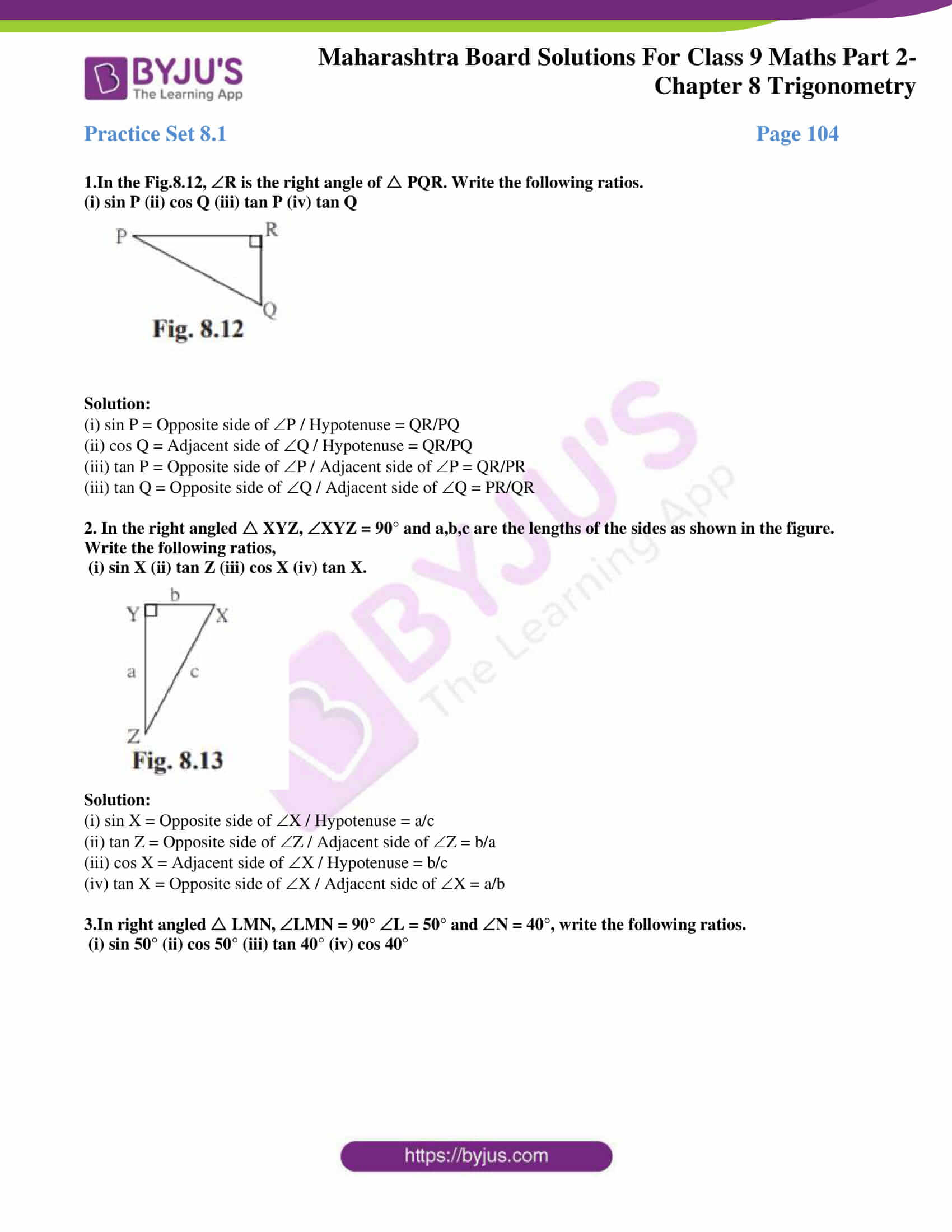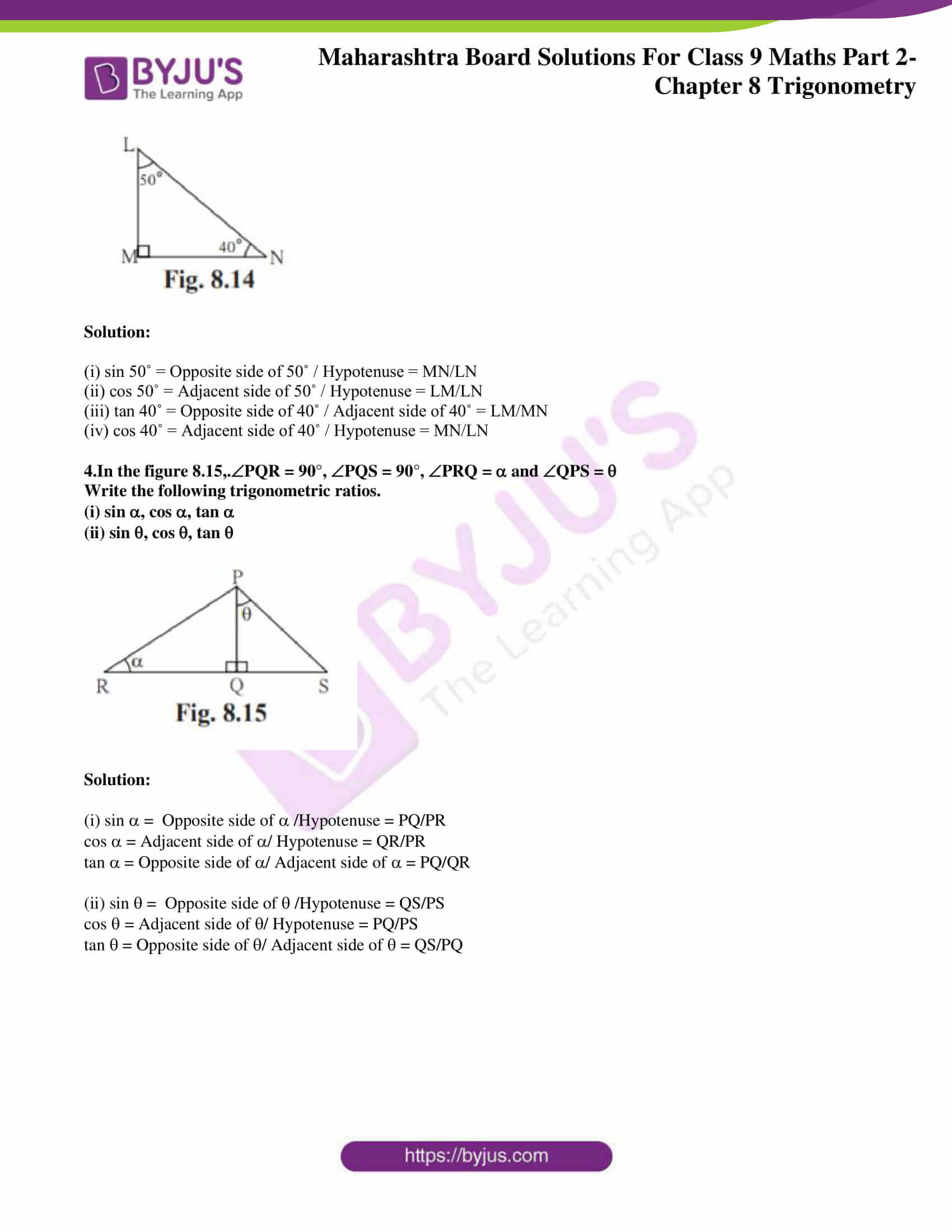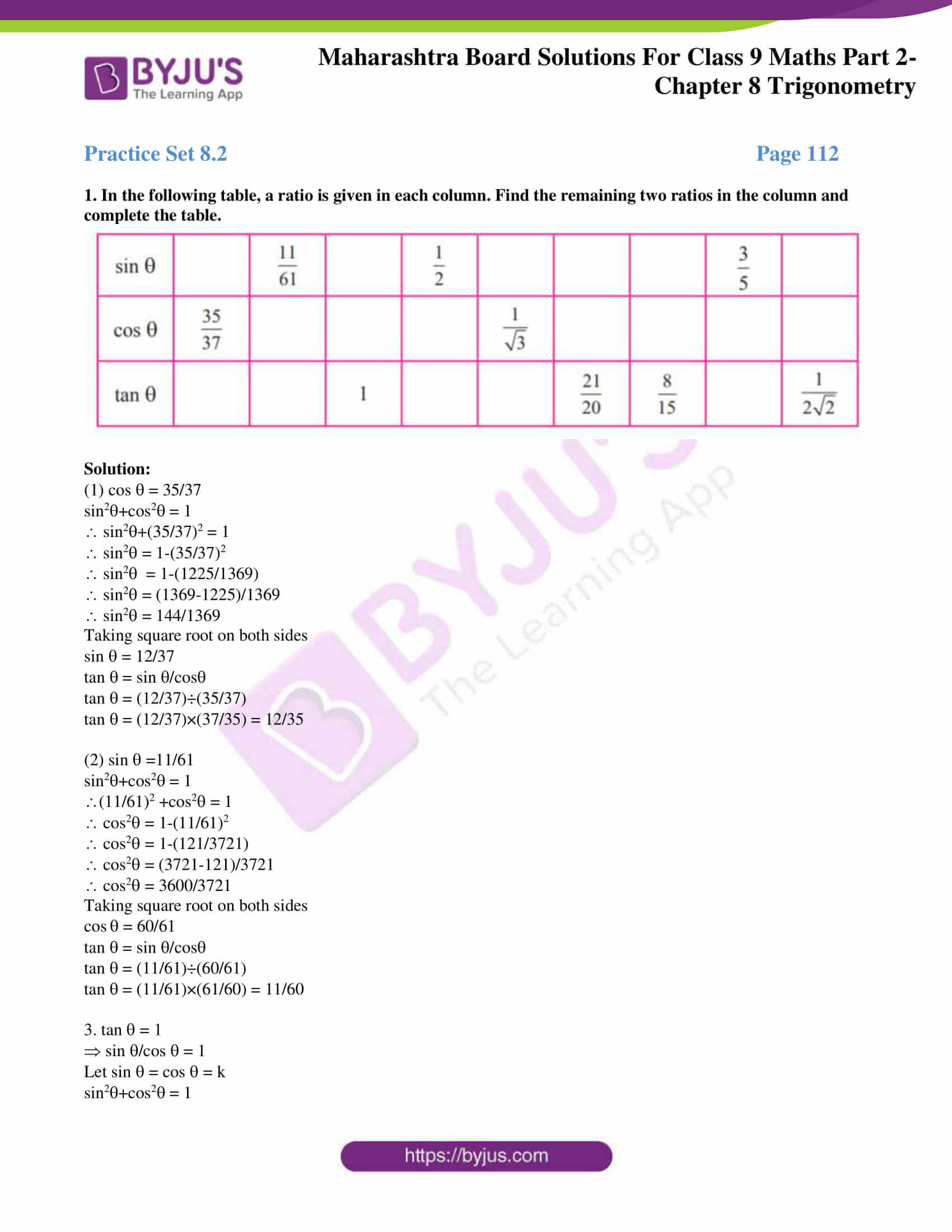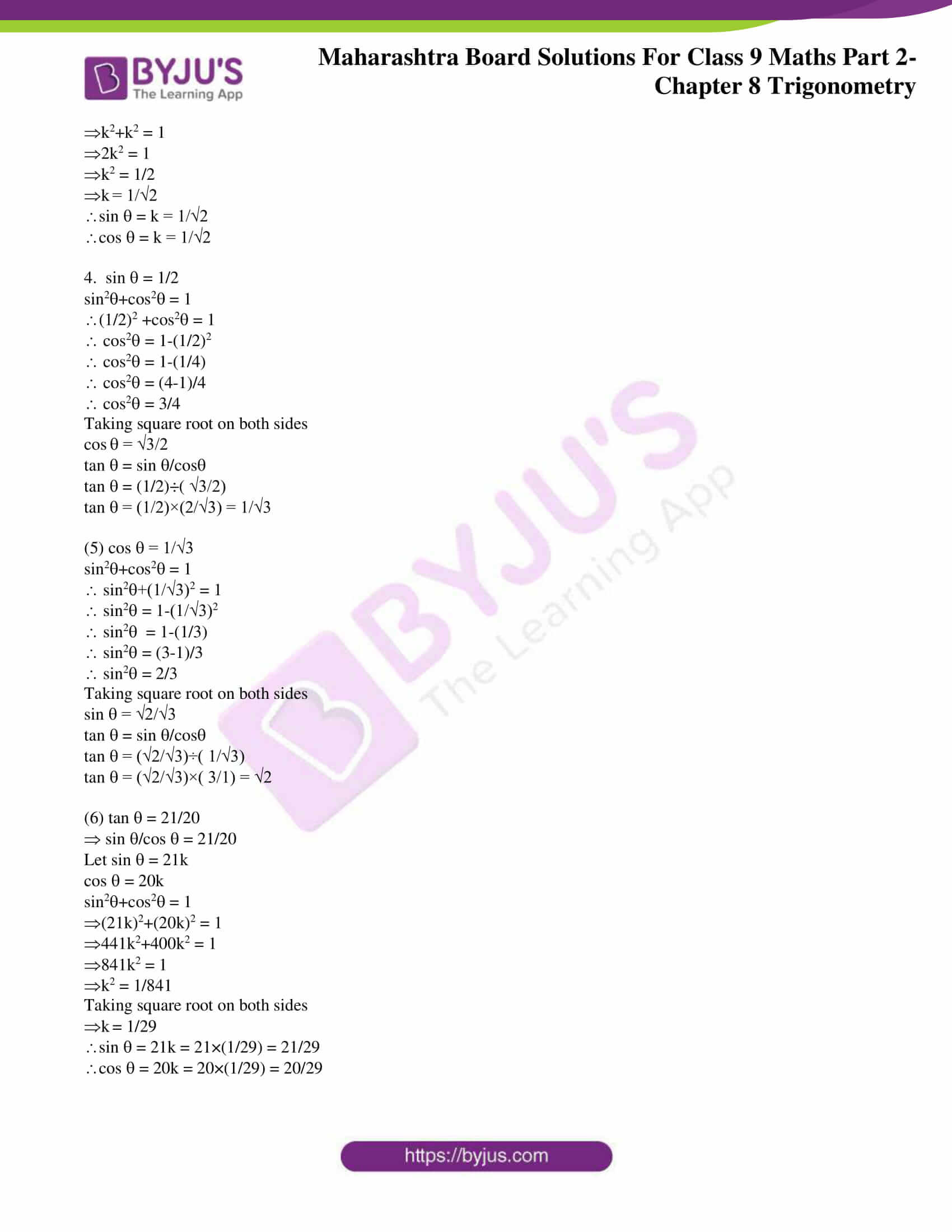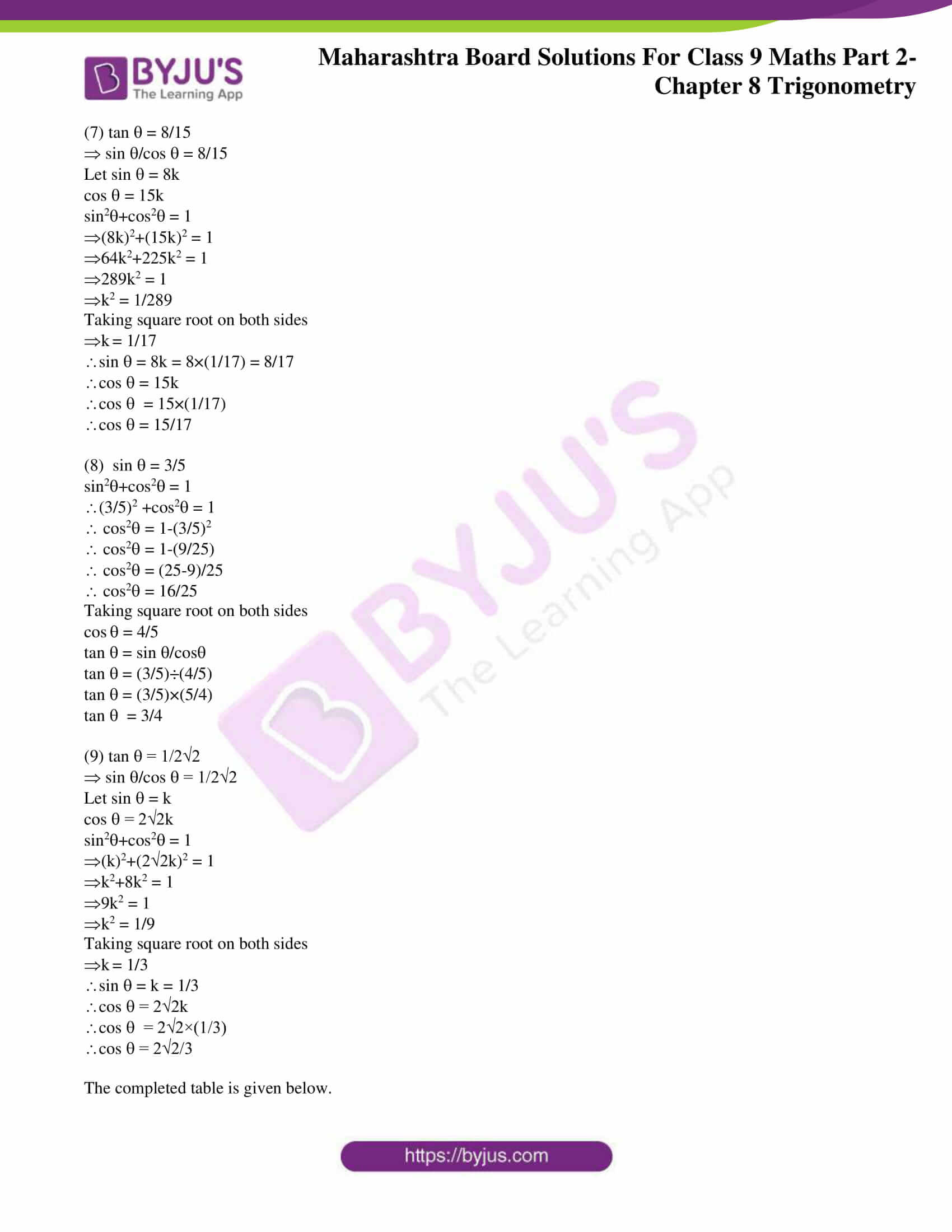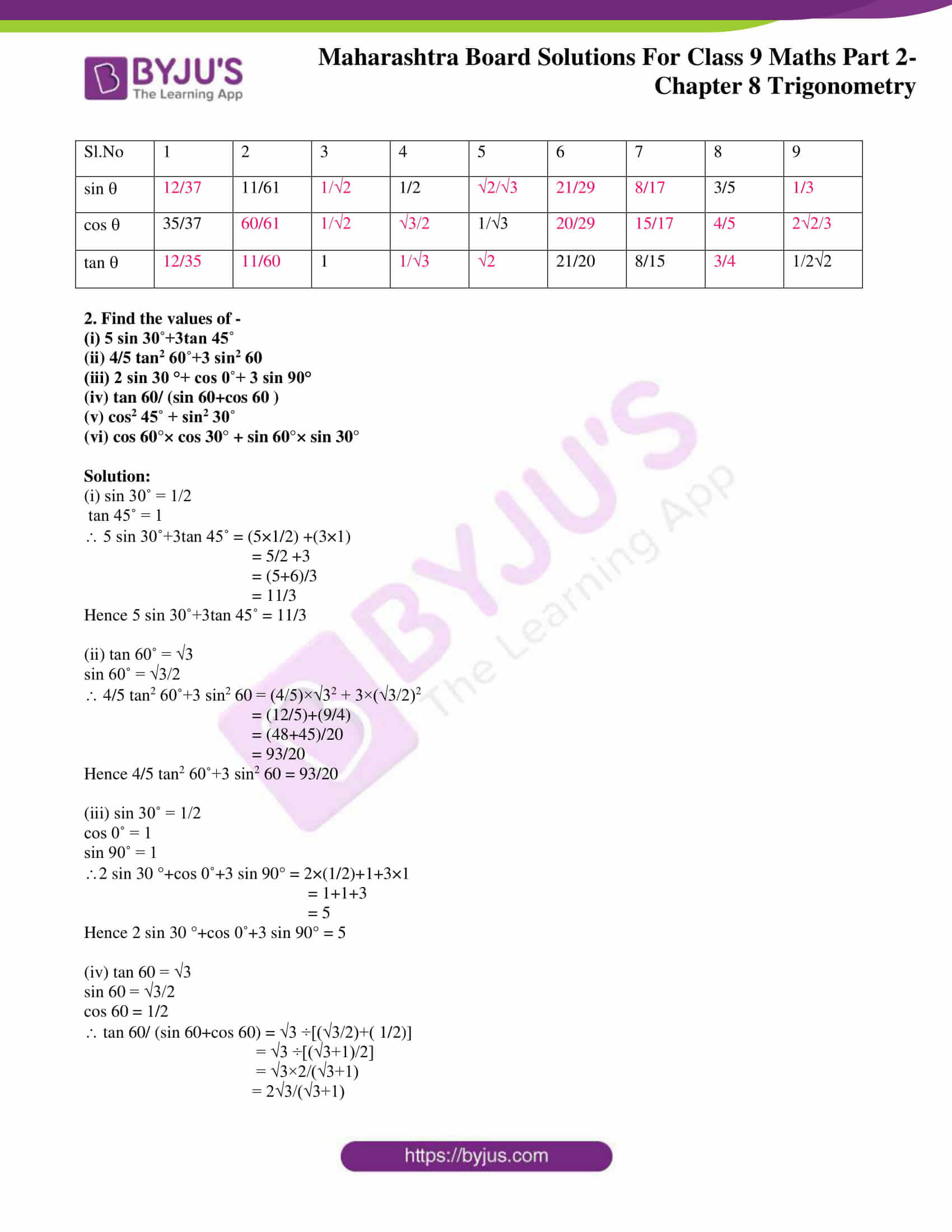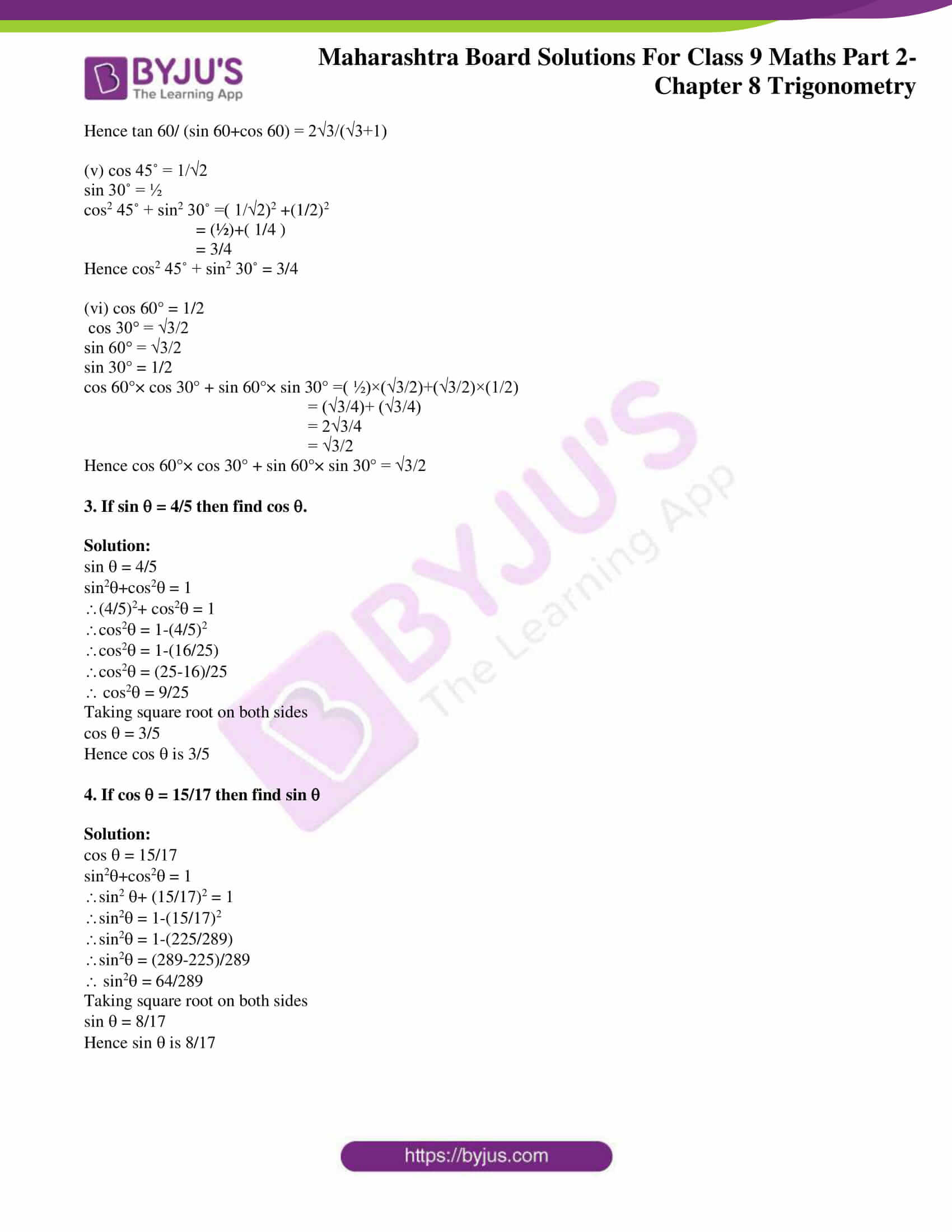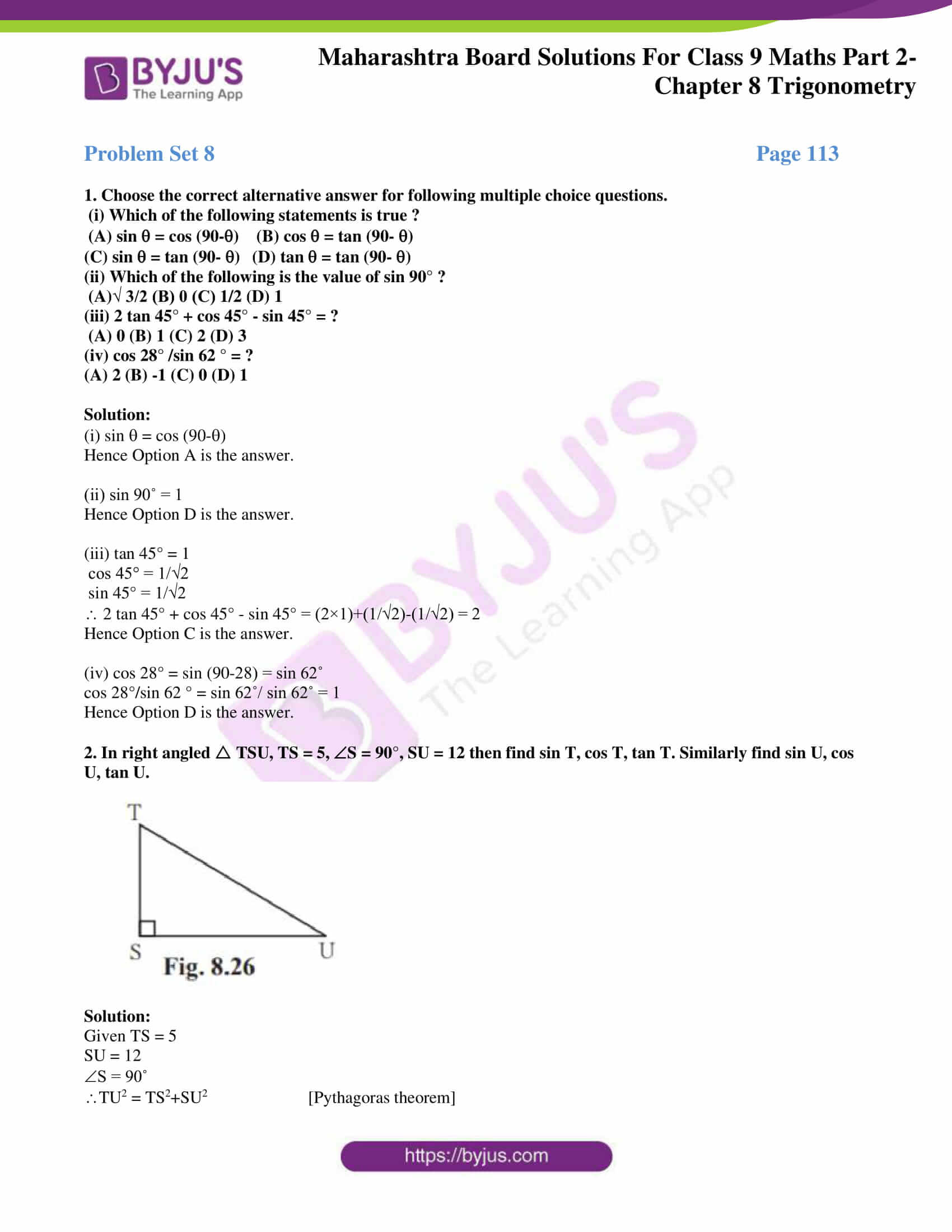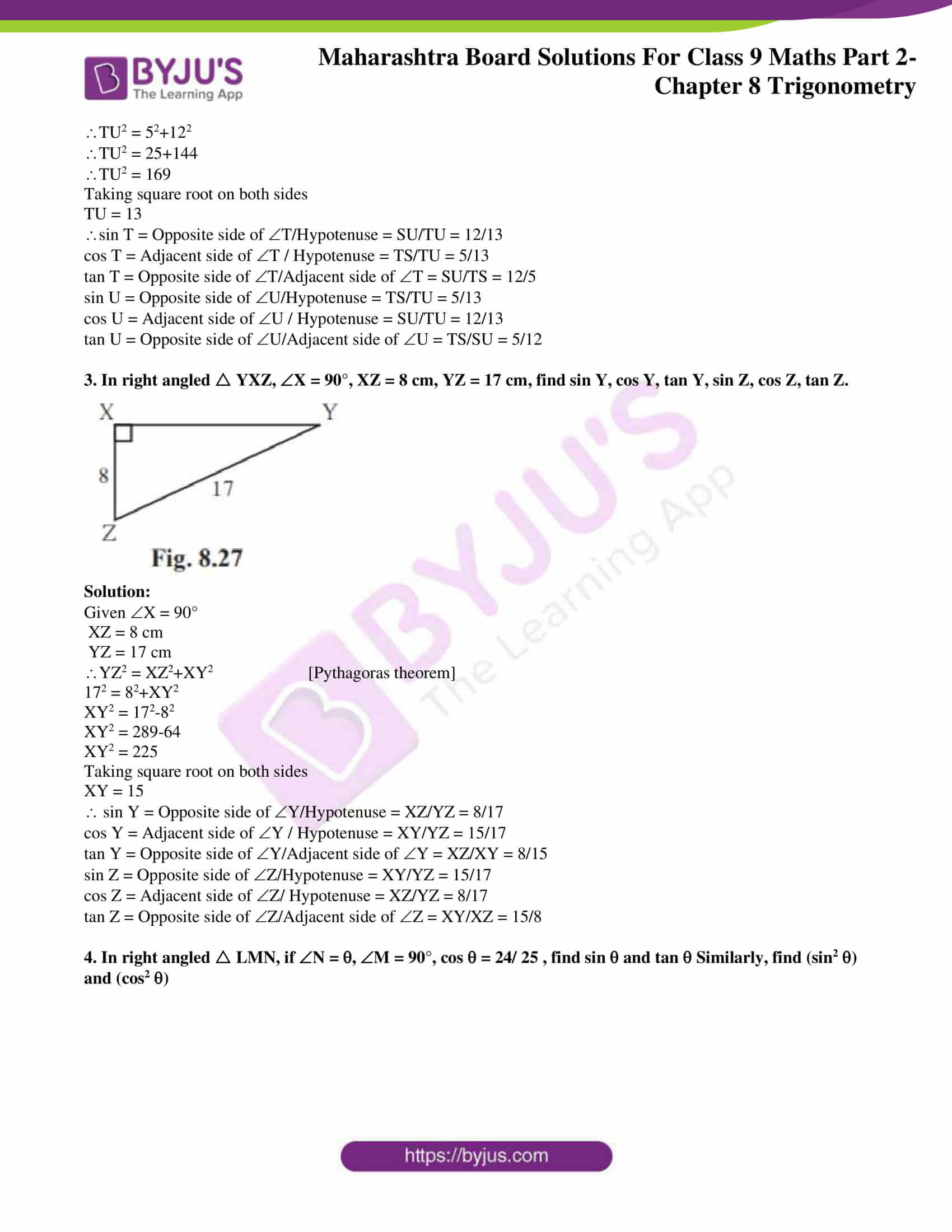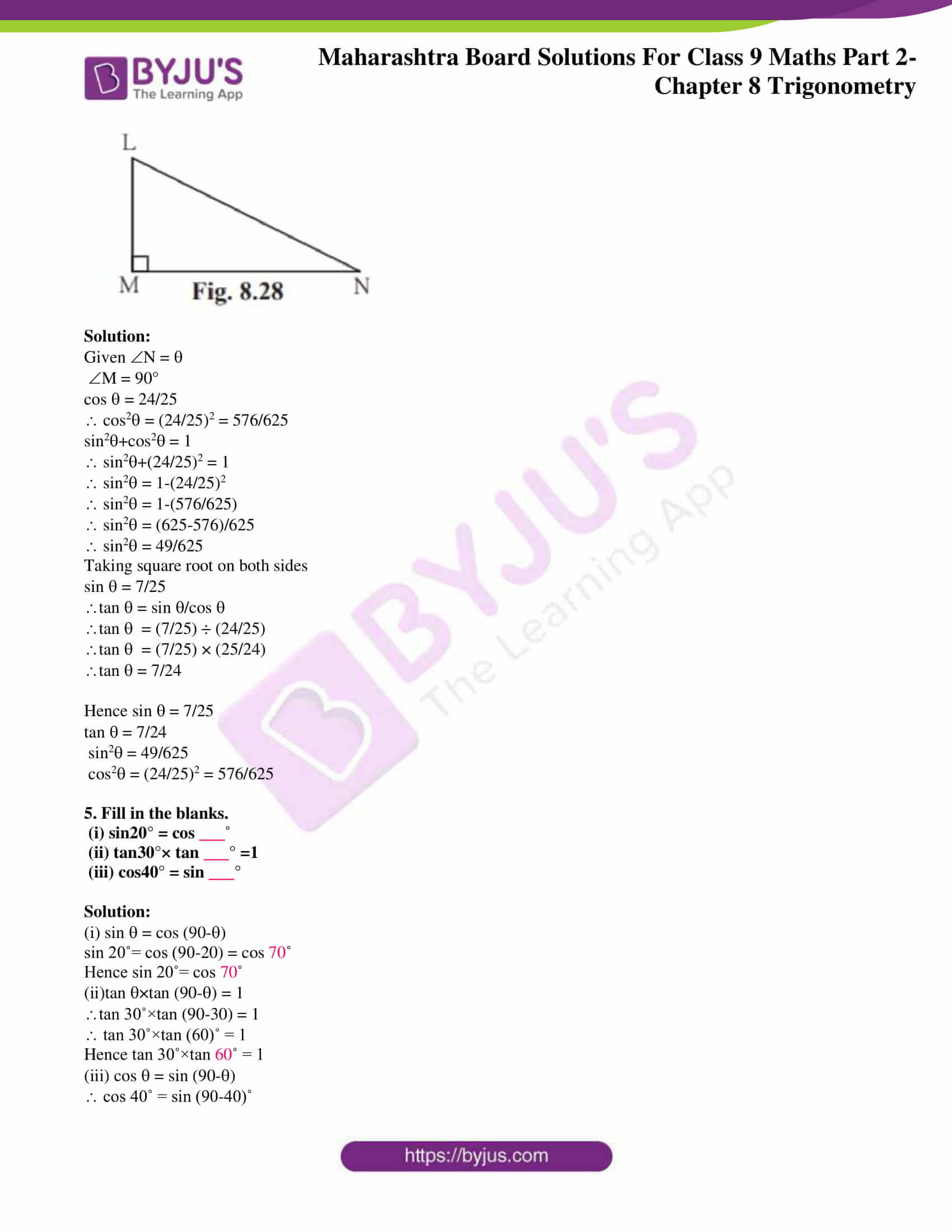## Access answers to Maths MSBSHSE Solutions For Class 9 Part 2 Chapter 8 – Trigonometry

Practice Set 8.1 Page 104

1.In the Fig.8.12, R is the right angle of PQR. Write the following ratios.

(i) sin P (ii) cos Q (iii) tan P (iv) tan Q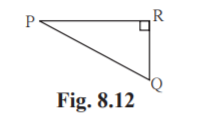Solution:

(i) sin P = Opposite side of P / Hypotenuse = QR/PQ

(ii) cos Q = Adjacent side of Q / Hypotenuse = QR/PQ

(iii) tan P = Opposite side of P / Adjacent side of P = QR/PR

(iii) tan Q = Opposite side of Q / Adjacent side of Q = PR/QR

2. In the right angled XYZ, XYZ = 90° and a,b,c are the lengths of the sides as shown in the figure. Write the following ratios,

(i) sin X (ii) tan Z (iii) cos X (iv) tan X.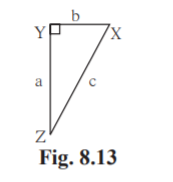Solution:

(i) sin X = Opposite side of X / Hypotenuse = a/c

(ii) tan Z = Opposite side of Z / Adjacent side of Z = b/a

(iii) cos X = Adjacent side of X / Hypotenuse = b/c

(iv) tan X = Opposite side of X / Adjacent side of X = a/b

3.In right angled LMN, LMN = 90° L = 50° and N = 40°, write the following ratios.

(i) sin 50° (ii) cos 50° (iii) tan 40° (iv) cos 40°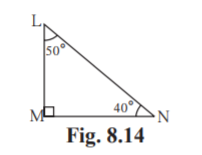Solution:

(i) sin 50˚ = Opposite side of 50˚ / Hypotenuse = MN/LN

(ii) cos 50˚ = Adjacent side of 50˚ / Hypotenuse = LM/LN

(iii) tan 40˚ = Opposite side of 40˚ / Adjacent side of 40˚ = LM/MN

(iv) cos 40˚ = Adjacent side of 40˚ / Hypotenuse = MN/LN

4.In the figure 8.15,.PQR = 90°, PQS = 90°, PRQ = and QPS =

Write the following trigonometric ratios.

(i) sin , cos , tan

(ii) sin , cos , tan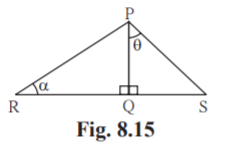Solution:

(i) sin = Opposite side of /Hypotenuse = PQ/PR

cos = Adjacent side of / Hypotenuse = QR/PR

tan = Opposite side of / Adjacent side of = PQ/QR

(ii) sin = Opposite side of /Hypotenuse = QS/PS

cos = Adjacent side of / Hypotenuse = PQ/PS

tan = Opposite side of / Adjacent side of = QS/PQ

Practice Set 8.2 Page 112

1. In the following table, a ratio is given in each column. Find the remaining two ratios in the column and complete the table.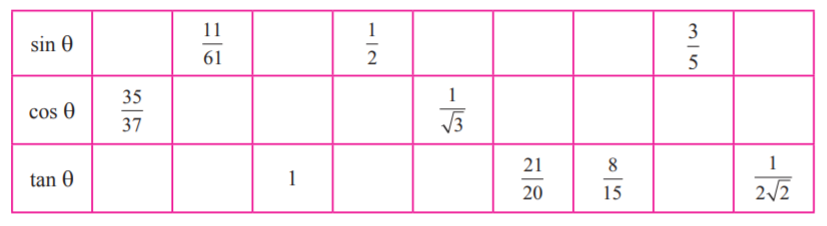Solution:

(1) cos = 35/37

sin2+cos2 = 1

sin2+(35/37)2 = 1

sin2 = 1-(35/37)2

sin2 = 1-(1225/1369)

sin2 = (1369-1225)/1369

sin2 = 144/1369

Taking square root on both sides

sin = 12/37

tan = sin /cos

tan = (12/37)÷(35/37)

tan = (12/37)×(37/35) = 12/35

(2) sin =11/61

sin2+cos2 = 1

(11/61)2 +cos2 = 1

cos2 = 1-(11/61)2

cos2 = 1-(121/3721)

cos2 = (3721-121)/3721

cos2 = 3600/3721

Taking square root on both sides

cos = 60/61

tan = sin /cos

tan = (11/61)÷(60/61)

tan = (11/61)×(61/60) = 11/60

3. tan = 1

sin /cos = 1

Let sin = cos = k

sin2+cos2 = 1

k2+k2 = 1

2k2 = 1

k2 = 1/2

k = 1/√2

sin = k = 1/√2

cos = k = 1/√2

4. sin = 1/2

sin2+cos2 = 1

(1/2)2 +cos2 = 1

cos2 = 1-(1/2)2

cos2 = 1-(1/4)

cos2 = (4-1)/4

cos2 = 3/4

Taking square root on both sides

cos = √3/2

tan = sin /cos

tan = (1/2)÷( √3/2)

tan = (1/2)×(2/√3) = 1/√3

(5) cos = 1/√3

sin2+cos2 = 1

sin2+(1/√3)2 = 1

sin2 = 1-(1/√3)2

sin2 = 1-(1/3)

sin2 = (3-1)/3

sin2 = 2/3

Taking square root on both sides

sin = √2/√3

tan = sin /cos

tan = (√2/√3)÷( 1/√3)

tan = (√2/√3)×( 3/1) = √2

(6) tan = 21/20

sin /cos = 21/20

Let sin = 21k

cos = 20k

sin2+cos2 = 1

(21k)2+(20k)2 = 1

441k2+400k2 = 1

841k2 = 1

k2 = 1/841

Taking square root on both sides

k = 1/29

sin = 21k = 21×(1/29) = 21/29

cos = 20k = 20×(1/29) = 20/29

(7) tan = 8/15

sin /cos = 8/15

Let sin = 8k

cos = 15k

sin2+cos2 = 1

(8k)2+(15k)2 = 1

64k2+225k2 = 1

289k2 = 1

k2 = 1/289

Taking square root on both sides

k = 1/17

sin = 8k = 8×(1/17) = 8/17

cos = 15k

cos = 15×(1/17)

cos = 15/17

(8) sin = 3/5

sin2+cos2 = 1

(3/5)2 +cos2 = 1

cos2 = 1-(3/5)2

cos2 = 1-(9/25)

cos2 = (25-9)/25

cos2 = 16/25

Taking square root on both sides

cos = 4/5

tan = sin /cos

tan = (3/5)÷(4/5)

tan = (3/5)×(5/4)

tan = 3/4

(9) tan = 1/2√2

sin /cos = 1/2√2

Let sin = k

cos = 2√2k

sin2+cos2 = 1

(k)2+(2√2k)2 = 1

k2+8k2 = 1

9k2 = 1

k2 = 1/9

Taking square root on both sides

k = 1/3

sin = k = 1/3

cos = 2√2k

cos = 2√2×(1/3)

cos = 2√2/3

The completed table is given below.

 Sl.No 1 2 3 4 5 6 7 8 9 sin 12/37 11/61 1/√2 1/2 √2/√3 21/29 8/17 3/5 1/3 cos 35/37 60/61 1/√2 √3/2 1/√3 20/29 15/17 4/5 2√2/3 tan 12/35 11/60 1 1/√3 √2 21/20 8/15 3/4 1/2√2

2. Find the values of –

(i) 5 sin 30˚+3tan 45˚

(ii) 4/5 tan2 60˚+3 sin2 60

(iii) 2 sin 30 °+ cos 0˚+ 3 sin 90°

(iv) tan 60/ (sin 60+cos 60 )

(v) cos2 45˚ + sin2 30˚

(vi) cos 60°× cos 30° + sin 60°× sin 30°

Solution:

(i) sin 30˚ = 1/2

tan 45˚ = 1

5 sin 30˚+3tan 45˚ = (5×1/2) +(3×1)

= 5/2 +3

= (5+6)/3

= 11/3

Hence, 5 sin 30˚+3tan 45˚ = 11/3

(ii) tan 60˚ = √3

sin 60˚ = √3/2

4/5 tan2 60˚+3 sin2 60 = (4/5)×√32 + 3×(√3/2)2

= (12/5)+(9/4)

= (48+45)/20

= 93/20

Hence, 4/5 tan2 60˚+3 sin2 60 = 93/20

(iii) sin 30˚ = 1/2

cos 0˚ = 1

sin 90˚ = 1

2 sin 30°+cos 0˚+3 sin 90° = 2×(1/2)+1+3×1

= 1+1+3

= 5

Hence, 2 sin 30°+cos 0˚+3 sin 90° = 5

(iv) tan 60 = √3

sin 60 = √3/2

cos 60 = 1/2

tan 60/ (sin 60+cos 60) = √3 ÷[(√3/2)+( 1/2)]

= √3 ÷[(√3+1)/2]

= √3×2/(√3+1)

= 2√3/(√3+1)

Hence tan 60/ (sin 60+cos 60) = 2√3/(√3+1)

(v) cos 45˚ = 1/√2

sin 30˚ = ½

cos2 45˚ + sin2 30˚ =( 1/√2)2 +(1/2)2

= (½)+(1/4)

= 3/4

Hence, cos2 45˚ + sin2 30˚ = 3/4

(vi) cos 60° = 1/2

cos 30° = √3/2

sin 60° = √3/2

sin 30° = 1/2

cos 60°× cos 30° + sin 60°× sin 30° =( ½)×(√3/2)+(√3/2)×(1/2)

= (√3/4)+ (√3/4)

= 2√3/4

= √3/2

Hence, cos 60°× cos 30° + sin 60°× sin 30° = √3/2

3. If sin = 4/5 then find cos.

Solution:

sin = 4/5

sin2+cos2 = 1

(4/5)2+ cos2 = 1

cos2 = 1-(4/5)2

cos2 = 1-(16/25)

cos2 = (25-16)/25

cos2 = 9/25

Taking square root on both sides

cos = 3/5

Hence, cos is 3/5

4. If cos = 15/17 then find sin

Solution:

cos = 15/17

sin2+cos2 = 1

sin2 + (15/17)2 = 1

sin2 = 1-(15/17)2

sin2 = 1-(225/289)

sin2 = (289-225)/289

sin2 = 64/289

Taking square root on both sides

sin = 8/17

Hence, sin is 8/17

Problem Set 8-Page 113

1. Choose the correct alternative answer for following multiple choice questions.

(i) Which of the following statements is true ?

(A) sin = cos (90-) (B) cos = tan (90-)

(C) sin = tan (90-) (D) tan = tan (90-)

(ii) Which of the following is the value of sin 90° ?

(A)√ 3/2 (B) 0 (C) 1/2 (D) 1

(iii) 2 tan 45° + cos 45° – sin 45° = ?

(A) 0 (B) 1 (C) 2 (D) 3

(iv) cos 28° /sin 62° = ?

(A) 2 (B) -1 (C) 0 (D) 1

Solution:

(i) sin = cos (90-)

Hence, Option A is the answer.

(ii) sin 90˚ = 1

Hence, Option D is the answer.

(iii) tan 45° = 1

cos 45° = 1/√2

sin 45° = 1/√2

2 tan 45° + cos 45° – sin 45° = (2×1)+(1/√2)-(1/√2) = 2

Hence Option C is the answer.

(iv) cos 28° = sin (90-28) = sin 62˚

cos 28°/sin 62 ° = sin 62˚/ sin 62˚ = 1

Hence Option D is the answer.

2. In right angled TSU, TS = 5, S = 90°, SU = 12 then find sin T, cos T, tan T. Similarly find sin U, cos U, tan U.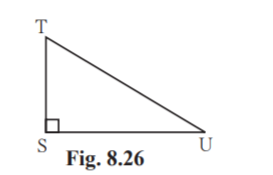Solution:

Given TS = 5

SU = 12

S = 90˚

TU2 = TS2+SU2 [Pythagoras theorem]

TU2 = 52+122

TU2 = 25+144

TU2 = 169

Taking square root on both sides

TU = 13

sin T = Opposite side of T/Hypotenuse = SU/TU = 12/13

cos T = Adjacent side of T / Hypotenuse = TS/TU = 5/13

tan T = Opposite side of T/Adjacent side of T = SU/TS = 12/5

sin U = Opposite side of U/Hypotenuse = TS/TU = 5/13

cos U = Adjacent side of U / Hypotenuse = SU/TU = 12/13

tan U = Opposite side of U/Adjacent side of U = TS/SU = 5/12

3. In right angled YXZ, X = 90°, XZ = 8 cm, YZ = 17 cm, find sin Y, cos Y, tan Y, sin Z, cos Z, tan Z.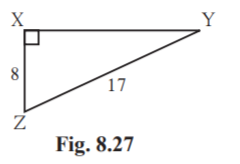Solution:

Given X = 90°

XZ = 8 cm

YZ = 17 cm

YZ2 = XZ2+XY2 [Pythagoras theorem]

172 = 82+XY2

XY2 = 172-82

XY2 = 289-64

XY2 = 225

Taking square root on both sides

XY = 15

sin Y = Opposite side of Y/Hypotenuse = XZ/YZ = 8/17

cos Y = Adjacent side of Y / Hypotenuse = XY/YZ = 15/17

tan Y = Opposite side of Y/Adjacent side of Y = XZ/XY = 8/15

sin Z = Opposite side of Z/Hypotenuse = XY/YZ = 15/17

cos Z = Adjacent side of Z/ Hypotenuse = XZ/YZ = 8/17

tan Z = Opposite side of Z/Adjacent side of Z = XY/XZ = 15/8

4. In right angled LMN, if N = , M = 90°, cos = 24/ 25 , find sin and tan Similarly, find (sin2 ) and (cos2 )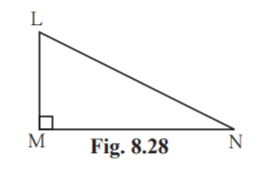Solution:

Given N =

M = 90°

cos = 24/25

cos2 = (24/25)2 = 576/625

sin2+cos2 = 1

sin2+(24/25)2 = 1

sin2 = 1-(24/25)2

sin2 = 1-(576/625)

sin2 = (625-576)/625

sin2 = 49/625

Taking square root on both sides

sin = 7/25

tan = sin /cos

tan = (7/25) ÷ (24/25)

tan = (7/25) × (25/24)

tan = 7/24

Hence sin = 7/25

tan = 7/24

sin2 = 49/625

cos2 = (24/25)2 = 576/625

5. Fill in the blanks.

(i) sin20° = cos ___˚

(ii) tan30°× tan ___° =1

(iii) cos40° = sin ___°

Solution:

(i) sin = cos (90-)

sin 20˚= cos (90-20) = cos 70˚

Hence, sin 20˚= cos 70˚

(ii)tan ×tan (90-) = 1

tan 30˚×tan (90-30) = 1

tan 30˚×tan (60)˚ = 1

Hence tan 30˚×tan 60˚ = 1

(iii) cos = sin (90-)

cos 40˚ = sin (90-40)˚

cos 40˚ = sin 50˚

Hence, cos 40˚ = sin 50˚

Trigonometry is a branch of Mathematics that deals with the relationship between side lengths and angles of triangles. This chapter explains the relations among trigonometric ratios and trigonometric ratios of particular angles. Sin, cos and tan are the three main trigonometric functions.

## Frequently Asked Questions on Maharashtra State Board Solutions for Class 9 Solutions Maths Part 2 Chapter 8 Trigonometry

### Will these Maharashtra State Board Solutions for Class 9 Solutions Maths Part 2 Chapter 8 Trigonometry be useful to prepare for the exams?

Students are highly recommended practising these solutions after revising the subjects, as they set the basis for the questions that could get asked often in the board exams. They act as the perfect guide for Class 9 students during their board exam preparation because it is created by qualified subject teachers according to the latest Class 9 Maths Syllabus. Students can discover various solved and unsolved questions and exercises that will aid them to prepare well for board exams.